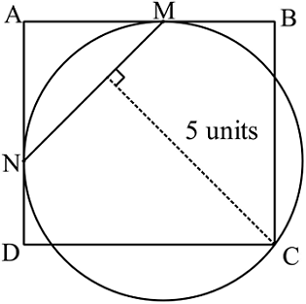# INMO Problem!

Geometry Level 3A circle passes through the vertex $C$ of a rectangle $ABCD$ and is tangent to the sides $AB$ and $AD$ at $M$ and $N$ respectively. If the distance from $C$ to the line segment $MN$ is equal to 5 units, find the area of the rectangle $ABCD$ in $\text{unit}^2.$

×